Ml aggarwal solutionsOur top 5% students will be awarded a special scholarship to Lido.
Set your child up for success with Lido, book a class today!

. (a) The figure (i) given below shows the cross-section of the concrete structure with the measurements

as given. Calculate the area of cross-section.

(b) The figure (ii) given below shows a field with the measurements given in meters. Find the area of the

field.

(c) Calculate the area of the pentagon ABCDE shown in fig (iii) below, given that AX = BX = 6 cm, EY =

CY = 4 cm, DE = DC = 5 cm, DX = 9 cm and DX is perpendicular to EC and AB.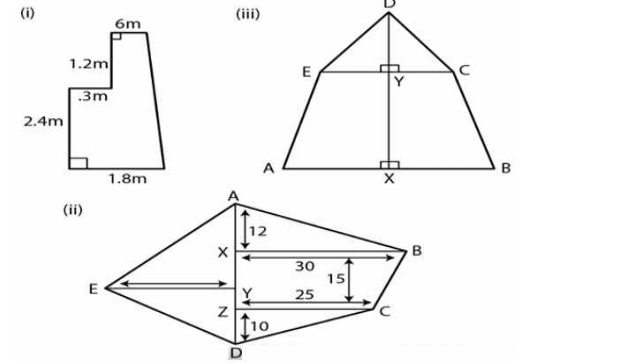Looking to do well in your science exam ? Learn from an expert tutor. Book a free class!

(a) From the figure (i)

AB = 1.8 m, CD = 0.6 m, DE = 1.2 m

EF = 0.3 m, AF = 2.4 m

Construct DE to meet AB in G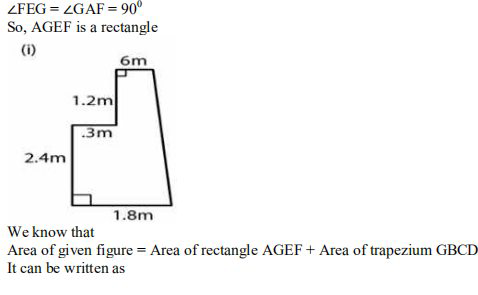= l × b + ½ (sum of parallel sides × height)

= AF × AG + ½ (GB + CD) × DG

Substituting the values

= 2.4 × 0.3 + ½ [(AB – AG) + CD] × (DE + EG)

Here AG = FE and using EG = AF

= 0.72 + ½ [(1.8 – 0.3) + 0.6] × (1.2 + 2.4)

By further calculation

= 0.72 + ½ [1.5 + 0.6] × 3.6

= 0.72 + ½ × 2.1 × 3.6

So we get

= 0.72 + 2.1 × 1.8

= 0.72 + 3.78

=4.5 \mathrm{m}^{2}

(b) It is given that

ABCD is a pentagonal field

AX = 12 m, BX = 30 m, XZ = 15 m, CZ = 25 m,

DZ = 10 m, AD = 12 + 15 + 10 = 37 m, EY = 20 m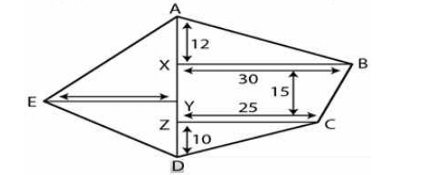We know that

Area of pentagonal field ABCDE = Area of triangle ABX + Area of trapezium BCZX + Area of triangle CDZ +

Area of triangle AED

It can be written as

= ½ × base × height + ½ (sum of parallel sides) × height + ½ × base × height + ½ × base × height

= ½ × BX × AX + ½ (BX + CZ) × XZ + ½ × CZ × DZ + ½ × AD × EY

Substituting the values

= ½ × 30 × 12 + ½ (30 + 25) × 15 + ½ × 25 × 10 + ½ × 37 × 20

By further calculation

= 15 × 12 + 7.5 × 55 + 25 × 5 + 37 × 10

So we get

= 180 + 412.5 + 125 + 370

=1087.5 \mathrm{m}^{2}

(c) It is given that

ABCDE is a pentagon

AX = BX = 6 cm, EY = CY = 4 cm

DE = DC = 5 cm, DX = 9 cm

Construct DX perpendicular to EC and AB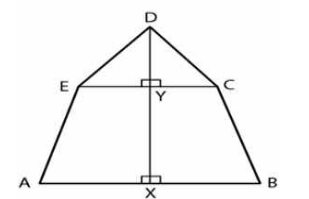In triangle DEY

Using Pythagoras Theorem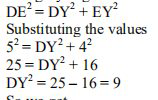DY = √9 = 3 cm

Here

Area of pentagonal field ABCDE = Area of triangle DEY + Area of triangle DCY + Area of trapezium EYXA +

Area of trapezium CYXB

It can be written as

= ½ × base × height + ½ × base × height + ½ × (sum of parallel sides) × height + ½ × (sum of parallel sides) ×

height

= ½ × EY × DY + ½ × CY × DY + ½ × (EY + AX) × XY + ½ × (CY + BX) × XY

Substituting the values

= ½ × 4 × 3 + ½ × 4 × 3 + ½ × (4 + 6) × (DX – DY) + ½ (4 + 6) × (DX – DY)

By further calculation

= 2 × 3 + 2 × 2 + ½ × 10 × (9 – 3) + ½ × 10 × (9 – 3)

So we get

= 6 + 6 + 5 × 6 + 5 × 6

= 6 + 6 + 30 + 30

=72 \mathrm{cm}^{2}

Our top 5% students will be awarded a special scholarship to Lido.Lido

Courses

Teachers

Book a Demo with us

Syllabus

Science
English
Coding

Terms & Policies

NCERT Question Bank

Maths
Science

Selina Question Bank

Maths
Physics
Biology

Allied Question Bank

Chemistry